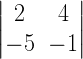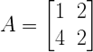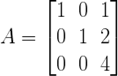NCERT Solutions for Maths Class 12 Exercise 4.1

# NCERT Solutions for Maths Class 12 Exercise 4.1

## Maths Class 12 Ex 4.1 Question 1.

Evaluate the following determinant:Solution:

## Maths Class 12 Ex 4.1 Question 2.

Solution:
(i)= cos θ × cos θ – (sin θ) × (–sin θ)
= cos² θ + sin² θ
= 1

## Maths Class 12 Ex 4.1 Question 3.

If, then show that |2A|= 4|A|

Solution:

## Maths Class 12 Ex 4.1 Question 4.

If, then show that |3A| = 27|A|

Solution:

## Maths Class 12 Ex 4.1 Question 5.

Evaluate the following determinants:

Solution:

## Maths Class 12 Ex 4.1 Question 6.

If, find |A|

Solution:

|A| == 1(9 + 12) 1(18 + 15) 2(8 5)
= 0

## Maths Class 12 Ex 4.1 Question 7.

Find the values of x, if

Solution:
(i)2 – 20 = 2x² – 24
x² = 3
x = ±√3

2 × 5 – 4 × 3 = 5 × x – 2x × 3

10 – 12 = 5x – 6x
x = 2

## Maths Class 12 Ex 4.1 Question 8.

If, then x is equal to

(A) 6
(B) ±6
(C) -6
(D) 0

Solution:
(B)x² – 36 = 36 – 36
x² = 36
x = ± 6

Hence, option (B) is correct.